## Thursday, December 18, 2008

### Today's Slides: December 18

Here they are ...

## Wednesday, December 17, 2008

### Solving Rational Equations

Hi! Today we had two visitors who monitored us in class. Mr. Kuropatwa went over yesterday’s work and also gave us new questions to work on. During the workshop, we has to reach upto 15 slides but we only got to about 9 slides. I will TRY my best to explain everything so you can understand it:)

First, we should all know how to multiply binomials by this time.

For example:And when its factored it looks like this:This should all be familiar to us.

When factoring trinomials, you get the ones at the top and when you reverse it you get multiplying binomials. It is like undoing the expressions.

Now Mr. K introduced us an easier and faster way to multiply two digit numbers.

For example:

41 x 31 = ?

First you multiply the two 1st digits

4x3 which equals to 12 then multiply the second digits which are1x1=1

so, so far you have 12 and 1. .leave a blank in between 12__ 1

now to find out what the blank is, you need to do a rainbown multiplication

the rainbow multiplication would be 1x3=3 and 4x1=4 and 3+4 = 7

the middle number would be 7.

The Fast Multiplication relates to the first part because of how the procedures are done.

Heres a picture that might help you understand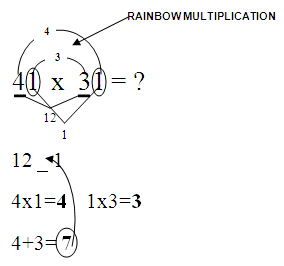Now the second part that we went over is balancing equations.

Ever since i have learned balancing equations form the past, I have

been transposing. .I guess it was the faster way of doing it and easier and I still get the right answer but today in class Mr. K. taught us something new. .

In this type of questions or any questions we DO NOT transpose because we don't just throw numbers from on side to another, we balance it.

to find the x. .

we make zero pairs so since there is x + 3 on the left side and 2 + 3x on the right side.

Add a negative x on the left side and add negative two on the right side.

Right now it isn't balanced so what we do on one side we do on the other

So we need to add -x and -2 on each side to balance the equation--there we go BALANCE EQUATIONS

The next question was. .First of all find the greatest common factor or GCF. . not LCD because we are dividing to get rid of the denominator.

In this case the gcf is 4 because 4 can be devided by 2 and 4 evenly. When you have found the gcf, you have to multiply it by every term to balance it.

On the first term 4÷2=2 so 2 stays there since it cannot be reduced anymore. Now on the last term, you can reduce 4÷4=1 so it is reduced. REM

EMBER! "REDUCE" not cancell

Now since everything has been reduced you can multiply the rest

Finally. .The gcf on this equation is x.

However ake sure to state the restrictions after finding the gfc.

Now multiply x by every term to balance it. On the first term the x's are going to reduce so you're left with a four.

Just multiply every term by x and you will be left with 4= -4x.

To get the x by itself, divide both sides by -4.JUST REMEMBER DO NOT FORGET TO STATE RESTRICTIONS OR ELSE YOU WILL LOSE A MARK !!!

Mr. K asked us if this was a rational equation, since it can be in fractions it is shown to be rational.

Please feel free to comment on this. Let me know if I have made any errors or if you want to share something and I'll add to it. If you still don't understand, talk to someone who knows best. Thanks and I hope you understand my scribe. .HAPPY HOLIDAYS!!!*

THE NEXT SCRIBE WILL BE ÿâssiÑⁿ♣ !!!!!!!!!!!

### Today's Slides: December 17

Here they are ...

## Tuesday, December 16, 2008

### Simplifying Rational Expressions

First of all we played with the Google translator. Woohoo!

The class was kind of slow today, we just did some questions on simplifying rational expressions.

Some notes:
• We don't do subtraction in High school, we add a negative number.
• Give everybody a home
• Expressions are different from Equations:
In an expression, the denominator remains while in an equation,
the denominator is cleared out.

This is one of the expressions Mr. Kuropatwa asked us to do:First, we factor it out.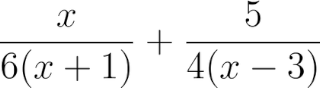Before we lose a mark, we should state the restrictions now.Then we find the common denominators which is: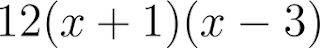Now we should multiply each expressions with the number one, in another form, and it should give us the common denominator "because we built it that way".,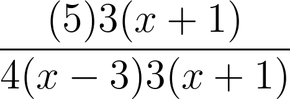Then we just add the numerators because we already have the common denominators.This would be the final result.But... in some expressions we could still further by factoring the numerator and reducing any common factors, but in this case we can't. Mr. K taught us a way to find out if we can still factor an expression, here's how:

We multiply the leading coefficient by the constant.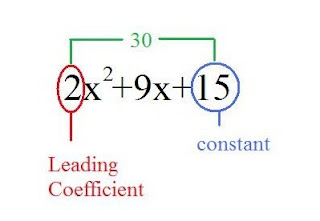Then we look for the factor of that number that will add up to 9 which is the middle term.
In this case we can't and that makes it a PRIME number!

That's all I could say so see yah!

And Levi would be the next scribe...

### Today's Slides: December 16

Here they are ...

## Monday, December 15, 2008

### Operations On Rational Expressions

On Today's class, we did workshop working on Rational Expressions. Mr. Kuropatwa gave us some examples that we need to work on in groups. After that, some of us in class explained how they got this answers.

Here are the examples that we worked on today...

a.)So first you need to factor the numerator and then factor the denominator to get the binomial.
So it would be look like....

Then.. you need to state what the restriction is.
So the restriction would be look like...

And last, reduce the fraction you got with the binomial.
So the same numerator and denominator will be reduced.So it looks like..

b.)

This expression is just the same as above, so find the factors to get the binomials.

And then, find the restriction again..
So the restriction will be...

And Last.. Reduce it... So the answer will be...c.)

This time, this expression is different.. So first you need to find the LCD(Least Common Denominator) So in this situation, the LCD is 15a³. So to find it you need to do this...

So it supposed to look like this...

And That's it.

d.)

The last question is different from the two expressions, but it is just the same from the third question because you need to factor the denominator to find the LCD.

You need to factor the denominator so it will look like like this....

You need to find the LCD of the the denominatorAfter you solve it, it would look like this...And then after you solve this.. you will get this answer:

This expression can be simplified furthermore:
After simplified...

This expression could be simplifed furthermore..
It would look like this...

Well I think this is where I will end my blog.. So I Hope you guys will get it now..
And the next scribe will be..... Jesnicz!!,,

### Today's Slides: December 15

Here they are ...

## Sunday, December 14, 2008

### Rational

Today's class, we did a workshop class working on rational expressions. Same thing were just practicing the rational expressions.factor the number,restrictions and reduce.We had a homework Exercise 51 do not do question 11.

Step 1: Factor the numerator and the denominator.

Step 2: Express each common factor pair in the numerator and denominator as 1.

Step 3: Simplify and state any restrictions on the variable.

Here are some examples:I Hope you guys understand it..

Well....The next scribe will be Jigsaw....Jan